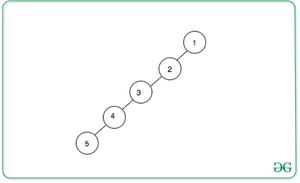GFG App
Open AppBrowser
Continue

# Check if a graph constructed from an array based on given conditions consists of a cycle or not

Given an array arr[] consisting of first N natural numbers, construct an undirected graph using the array elements such that for any array element, connect an edge with the next greater element on the left as well as right.

Examples:

Input: arr = {1, 2, 3, 4, 5}
Output: No
Explanation:
It is clear from the below image that final graph will be a tree.Input: arr[] = {1, 4, 2, 5, 3}
Output: Yes

Naive Approach: The simplest approach is to construct the required graph using the above conditions and check if there exists any cycle of at least length 3 or not. If there exists a cycle, then print “Yes“. Otherwise, print “No“.
Time Complexity: O(N + E), where E is the number of edges.
Auxiliary Space: O(N)

Efficient Approach: The optimal idea is to check if the given permutation is unimodal or non-unimodal, i.e.simply check if there exists any array element with greater adjacent elements on both sides. If found to be true, print “Yes”. Otherwise, print “No”.
Below is the implementation of the above approach:

## C++

 `// C++ program for the above approach`   `#include ` `using` `namespace` `std;`   `// Function to check if the graph` `// constructed from given array` `// contains a cycle or not` `void` `isCycleExists(``int` `arr[], ``int` `N)` `{` `    ``bool` `valley = 0;`   `    ``// Traverse the array` `    ``for` `(``int` `i = 1; i < N; i++) {`   `        ``// If arr[i] is less than` `        ``// arr[i - 1] and arr[i]` `        ``if` `(arr[i] < arr[i - 1]` `            ``&& arr[i] < arr[i + 1]) {`   `            ``cout << ``"Yes"` `<< endl;` `            ``return``;` `        ``}` `    ``}`   `    ``cout << ``"No"``;` `}`   `// Driver Code` `int` `main()` `{` `    ``// Given array` `    ``int` `arr[] = { 1, 3, 2, 4, 5 };`   `    ``// Size of the array` `    ``int` `N = ``sizeof``(arr)` `            ``/ ``sizeof``(arr);`   `    ``isCycleExists(arr, N);`   `    ``return` `0;` `}`

## Java

 `// Java program for the above approach` `import` `java.io.*;` `class` `GFG` `{`   `  ``// Function to check if the graph` `  ``// constructed from given array` `  ``// contains a cycle or not` `  ``static` `void` `isCycleExists(``int``[] arr, ``int` `N)` `  ``{`   `    ``// Traverse the array` `    ``for` `(``int` `i = ``1``; i < N; i++)` `    ``{`   `      ``// If arr[i] is less than` `      ``// arr[i - 1] and arr[i]` `      ``if` `(arr[i] < arr[i - ``1``]` `          ``&& arr[i] < arr[i + ``1``])` `      ``{` `        ``System.out.println(``"Yes"``);` `        ``return``;` `      ``}` `    ``}` `    ``System.out.println(``"No"``);` `  ``}`   `  ``// Driver Code` `  ``public` `static` `void` `main(String[] args)` `  ``{`   `    ``// Given array` `    ``int``[] arr = { ``1``, ``3``, ``2``, ``4``, ``5` `};`   `    ``// Size of the array` `    ``int` `N = arr.length;` `    ``isCycleExists(arr, N);` `  ``}` `}`   `// This code is contributed by Dharanendra L V.`

## Python3

 `# Python3 program for the above approach`   `# Function to check if the graph` `# constructed from given array` `# contains a cycle or not` `def` `isCycleExists(arr, N):` `    ``valley ``=` `0`   `    ``# Traverse the array` `    ``for` `i ``in` `range``(``1``, N):`   `        ``# If arr[i] is less than` `        ``# arr[i - 1] and arr[i]` `        ``if` `(arr[i] < arr[i ``-` `1``] ``and` `arr[i] < arr[i ``+` `1``]):` `            ``print``(``"Yes"``)` `            ``return` `    ``print``(``"No"``)`   `# Driver Code` `if` `__name__ ``=``=` `'__main__'``:` `  `  `    ``# Given array` `    ``arr ``=` `[``1``, ``3``, ``2``, ``4``, ``5``]`   `    ``# Size of the array` `    ``N ``=` `len``(arr)` `    ``isCycleExists(arr, N)`   `# This code is contributed by mohit kumar 29`

## C#

 `// C# program for the above approach` `using` `System;` `class` `GFG` `{`   `  ``// Function to check if the graph` `  ``// constructed from given array` `  ``// contains a cycle or not` `  ``static` `void` `isCycleExists(``int``[] arr, ``int` `N)` `  ``{`   `    ``// Traverse the array` `    ``for` `(``int` `i = 1; i < N; i++)` `    ``{`   `      ``// If arr[i] is less than` `      ``// arr[i - 1] and arr[i]` `      ``if` `(arr[i] < arr[i - 1]` `          ``&& arr[i] < arr[i + 1]) ` `      ``{` `        ``Console.WriteLine(``"Yes"``);` `        ``return``;` `      ``}` `    ``}` `    ``Console.WriteLine(``"No"``);` `  ``}`   `  ``// Driver Code` `  ``public` `static` `void` `Main()` `  ``{` `    `  `    ``// Given array` `    ``int``[] arr = { 1, 3, 2, 4, 5 };`   `    ``// Size of the array` `    ``int` `N = arr.Length;` `    ``isCycleExists(arr, N);` `  ``}` `}`   `// This code is contributed by chitranayal.`

## Javascript

 ``

Output:

`Yes`

Time Complexity: O(N)
Auxiliary Space: O(1)

My Personal Notes arrow_drop_up